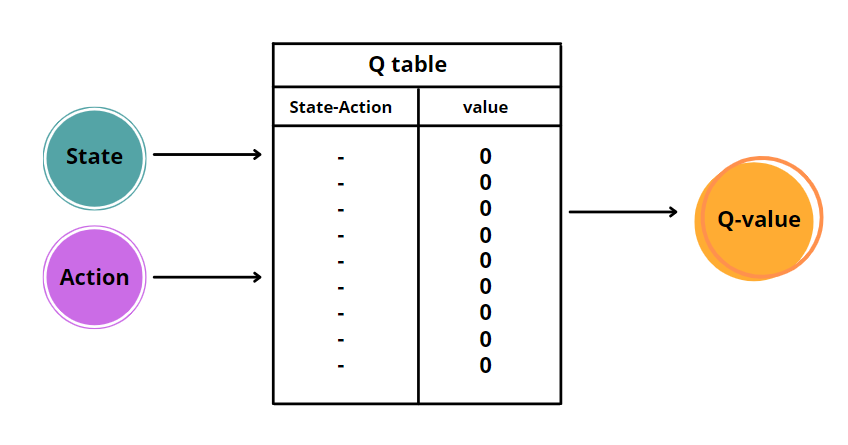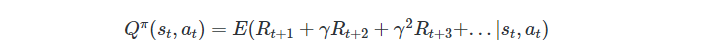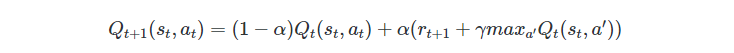Eugenia Anello — June 2, 2021

This article was published as a part of the Data Science Blogathon

## Overview

• Introduction to a classic Reinforcement Learning problem, Acrobot
• RL concepts covered – agent, environment, states, action, reward, Q-learning, Deep Q Learning
• Learn theory through examples and illustrations

Introduction

Acrobot is a game, in which a robotic arm is composed of two joints and two links and the joint between the two links is actued. In the beginning, the links are hanging downwards. The goal of the task is to move the end of the lower link up to a given height .

Below, you can see the game before and after training. The agent has no prior knowledge of how its actions affect the environment and learns by itself playing the game many times. In the beginning, the agent doesn’t know how to behave and within the time it will be able to understand how to act optimally.

In this post, I will show the principal concepts of Reinforcement Learning describing this game. What is Reinforcement Learning? It’s a branch of machine learning inspired by human behavior, how we learn interacting with the world. This field is widely applied for playing computer games and robotics. So, this game I am showing fits perfectly to understand deeply the concepts of DL.

There are five important concepts when you want to grasp reinforcement learning’s mentality. Agent, Environment, State, Action, and Reward. They are all linked to each other. And at the end, I will explain Deep Q learning, a powerful method that merges Deep Learning Techniques with RL.

## States

The state is the current condition of the robotic arm. The state can be constituted by the screen pixels of the game or by some informations about the agent, which can also be called environment observations. These lasts are:

• sin and cos of the two rotational joint angles (4 in total)
• the two angular velocities

In this post, I will focus on this external information, instead of the current frame.

## Actions and Rewards

When the robotic arm chooses and performs an action, it may receive a reward or not. In each time step, it receives -1 as punishment until the goal is reached. Then, the agent’s task is to understand which actions can maximize the cumulative reward. There are three possible actions:

• Apply positive torque (+1)
• Apply negative torque (-1)
• Do nothing (0)

## Q-learningQ-learning is an algorithm that quantifies the expected discounted future rewards that can be obtained by taking a certain action in a given state at any time step t. Then, it estimates the value of each state-action pair. Why Q? Q stands for Quality, which depends on how the action is useful to gain some reward in the future . The agent learns to associate to each state-action pair the Q-value, using the Bellman equation:Each step of the Q-learning algorithm is defined by the following equation:Don’t worry. I know, it scares you as soon as your eyes encounter this equation. We only need to understand the role of each parameter involved.

• s = state
• a = action
• r = reward
• t = time step
• γ = discount rate
• α = learning rate

Both the learning rate and discount rate are between 0 and 1. The last determines how much we care about the future reward. The more it’s near 1, the more we care.

The drawback of Q-learning is that it has issues with huge state and action spaces. Memorize every possible couple of actions and states needs big memory. For this reason, we need to combine Q-learning with Deep Learning techniques.

## Deep Q learning

As we saw, the Q-learning algorithm needs function approximators, such as artificial neural networks, to memorize the triplets (state, action, Q-value). The idea of Deep Q learning is to use neural networks to predict the Q-values for each action given the state. If we consider again the Acrobot game, we pass it to the artificial neural network as input the information is about the agent (sin and cos of joint angles, velocities). To obtain the predictions, we need to train the network before and define the loss function, which is usually the difference between the predicted Q-value and the target Q-value.

Different from supervised learning, we don’t have any label, that identifies the correct Q-value for each state-action pair. In DQL, we initialize two identical artificial neural networks, called Target Network and Policy Network. The first will be used to calculate the target values, while the second to determine the prediction.

For example, the model for the Acrobat’s game is an artificial neural network that takes into input the environment observations, the sin and cos of the two rotational joint angles, and the two angular velocities. It returns three outputs, Q(s,+1), Q(s,-1), Q(s,0), where s is the state, passed as input to the network. Indeed, the goal of the neural network is to predict the expected return of taking each action given the current input.

## Experience Replay

The ANN is not enough alone. Experience is a technique where we store the past data discovered by the agent for (state, action, reward, next state) at each time step. Later, we sample randomly the memory for a mini-batch of experience and we use it to train the artificial neural network. By sampling randomly, we allow to provide uncorrelated data to the neural network model and improve the efficiency of the data.

## Exploration vs Exploitation

Exploration and Exploitation are key concepts in the Deep RL algorithm. It refers to the way the agent selects the actions. What are Exploration and Exploitation? Let’s suppose that we want to go to a restaurant. Exploration is when you want to try a new restaurant, while exploitation is when you want to remain in your comfort zone, so you’ll go directly to your favorite restaurant. It’s the same for the agent. In the beginning, it wants to explore the environment. As long as it interacts with the environment, it will take choice more based on exploitation than exploration.

There are two possible strategies:

• ε-greedy, where the agent takes a random action with probability ε, then it explores the environment and selects the greedy action with probability 1-ε, then we are in an exploitation situation.
• soft-max, where the agent selects the optimal actions based on the Q-values returned by the artificial neural network.

## Final thoughts:

Congratulations! Now you grasp the RL and DRL concepts through the example of Acrobot that introduced you to this new world. Deep Q learning has gained a lot of attention after the applications in Atari games and Go. I hope that this guide didn’t scare you and will encourage you to go deeper into the topic. Thanks for reading. Have a nice day!

References:

 https://gym.openai.com/envs/Acrobot-v1/

 http://www.henrypan.com/blog/reinforcement-learning/2019/12/03/acrobot.html

The media shown in this article are not owned by Analytics Vidhya and are used at the Author’s discretion.